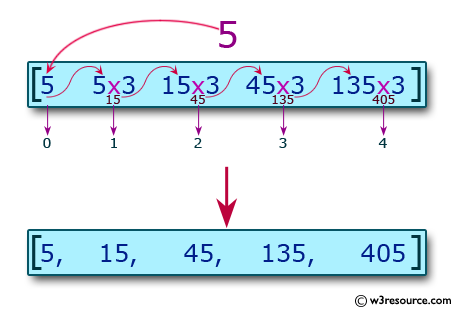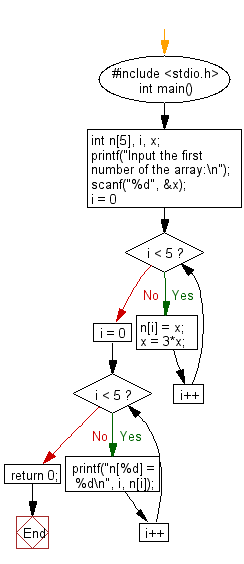﻿ C : Read and print the elements of an array of length 7

# C Exercises: Put the triple of the previous position starting from the second position of the array

## C Basic Declarations and Expressions: Exercise-49 with Solution

Write a C program to read and print the elements of an array with length 7. Before printing, insert the triple of the previous position, starting from the second position.
For example, if the first number is 2, the array numbers must be 2, 6, 18, 54 and 162Sample Solution:

C Code:

``````#include <stdio.h>
int main() {
int n, i, x;

// Input the first number of the array
printf("Input the first number of the array:\n");
scanf("%d", &x);

// Fill the array with values based on the input
for(i = 0; i < 5; i++) {
n[i] = x;
x = 3 * x;
}

// Print the array values
for(i = 0; i < 5; i++) {
printf("n[%d] = %d\n", i, n[i]);
}

return 0;
}
``````

Sample Output:

```Input the first number of the array:
5
n = 5
n = 15
n = 45
n = 135
n = 405
```

Flowchart:C programming Code Editor:

What is the difficulty level of this exercise?

Test your Programming skills with w3resource's quiz.

﻿